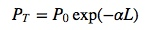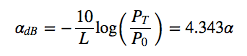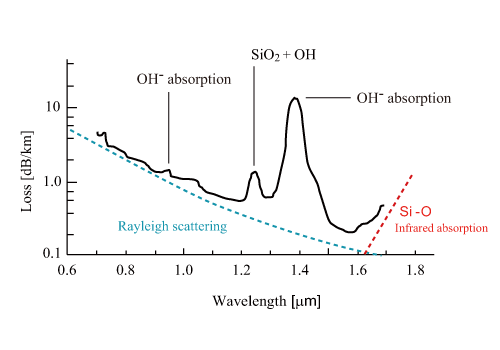When light propagates in optical fiber, the optical power loss occurs. The power loss is an important parameter for characterizing a fiber. Provided that light at the optical power of P0 is incident to an optical fiber with a length of L, the optical power after propagation in the fiber, PT, is expressed by the following equation,where α is the damping constant, representing loss in the optical fiber. The loss of optical fiber, α [dB/km], is generally given below.Fig.2.12 shows the wavelength dependency of fiber loss for a typical quartz optical fiber . The fiber loss, αdB, takes the minimum value of ca. 0.2[dB/km] (damping constant α≅4.6×10−7cm−1) at the wavelength of ca. 1.55 μm. The loss spectrum is determined by several factors. The primary factors are the absorption and the Rayleigh scattering by the fiber material itself. The effect of Rayleigh scattering is relatively larger for a shorter wavelength of light. The Rayleigh scattering loss in the visible range is 1∼10[dB/km], which is relatively smaller compared with a loss in other materials. Pure quartz glass has absorption bands only in the range longer than 2 μm and in the UV range. However, when an even slight amount of impurity is added, the quartz glass has strong absorptions in the range of 0.5∼2 μm, which is transmissive for the pure quartz glass.Figure 2.12: Wavelength dependency of loss in silica optical fiber

In the following sections, the primary factors of optical fiber loss are explained with categorizing them into the loss attributable to material characteristics, the loss by impurity absorption, and the loss by structural imperfection.